# Collecting & Analyzing Data on an Android Device

Companion for Android

### StatCalc Epidemiologic Calculators

The StatCalc tool is a calculator capable of analysis pre and post data collection. The version available on the Companion for Android has similar functionality to the full Epi Info™ StatCalc tool and is capable of performing the analyses listed in the StatCalc menu listed below.   For additional information regarding StatCalc, refer to the StatCalc chapter of the user guide.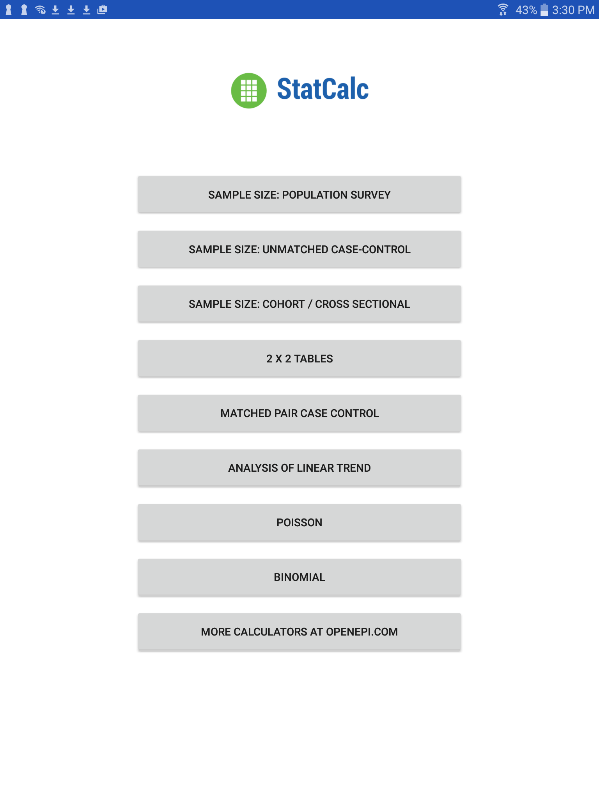### Sample Size: Population Survey

The population survey calculates how many samples are recommended for a survey given a population size, expected frequency, design effect, the number of clusters and the desired confidence level.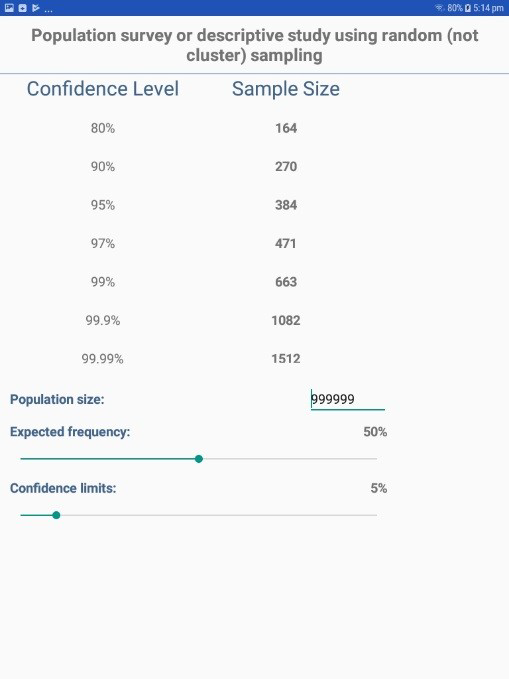Figure 6.71: Population Survey

### Sample Size: Unmatched Case-Control

The Unmatched Case Control study calculates how many samples are recommended for a study given the power, proportion of unexposed vs. exposed, percentage outcome in exposed group, percentage outcome in the unexposed group, and the desired confidence level.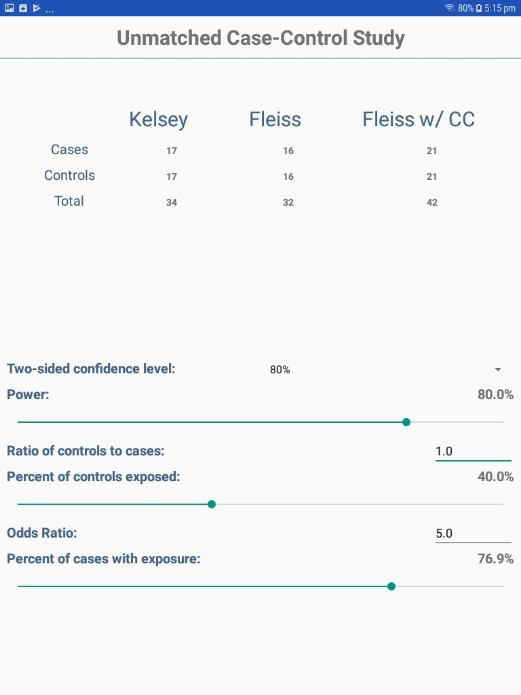Figure 6.72: Unmatched Case-Control

### Sample Size: Cohort / Cross Sectional

The Unmatched Cohort / Cross Section study calculates the sample size requirements for two groups that meet a set of research conditions: one group has a certain outcome (e.g., ill) or condition and other does not (e.g., not ill) based on a set of factors chosen by the researcher (e.g. confidence level, power (1 – α), ratio of exposed to unexposed, risk ratio, odds ratio, etc).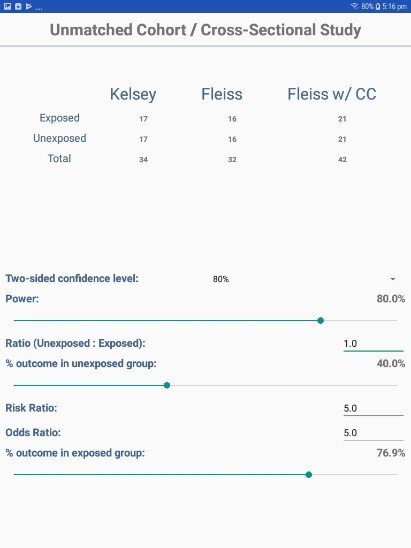Figure 6.73: Cohort/Cross Sectional

### Analysis of Single and Stratified Tables

Two by two tables are frequently used in epidemiology to explore associations between exposures to risk factors and disease or other outcomes. The table in StatCalc has Exposure on the left and Outcome across the top. Given a yes-no or other two-choice response describing disease and another describing exposure to a risk factor, StatCalc produces several kinds of statistics that test for relationships between exposure and disease.

Stratifying a dataset separates the population into distinct categories based on a parameter (i.e. sex). If confounding is present, associations between disease and exposure can be missed or falsely detected.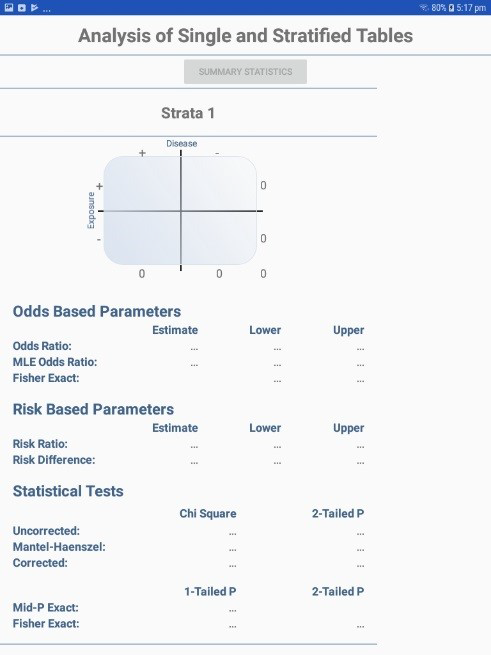Figure 6.74: Single and Stratified Tables

### Matched Pair Case-Control

The Matched Pair Case-Control Study calculates the statistical relationship between exposures and the likelihood of becoming ill in a given patient population. This study is used to investigate a cause of an illness by selecting a non-ill person as the control and matching the control to a case. The control can be matched to one or more criteria.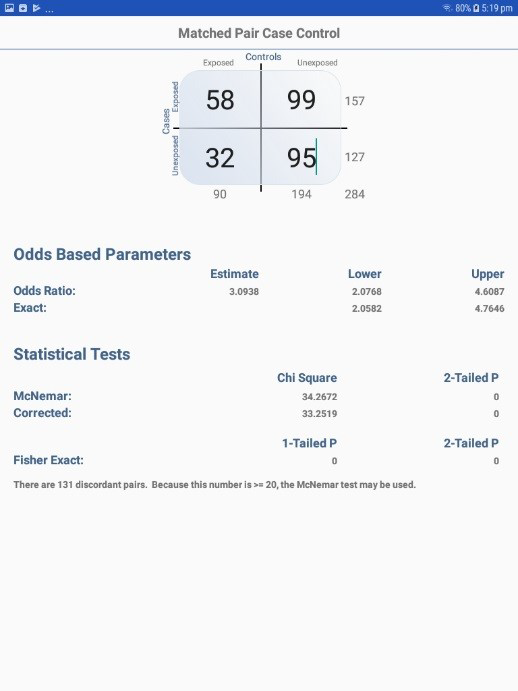Figure 6.75: Match Pair Case Control

### Analysis of Linear Trend

The Linear Trend function calculates the odds ratio, chi square for linear trend, and p-value statistics based on the response to an exposure score and whether or not the patient has become ill. The exposure score is a measured outcome from a study that states the level of exposure the patient received.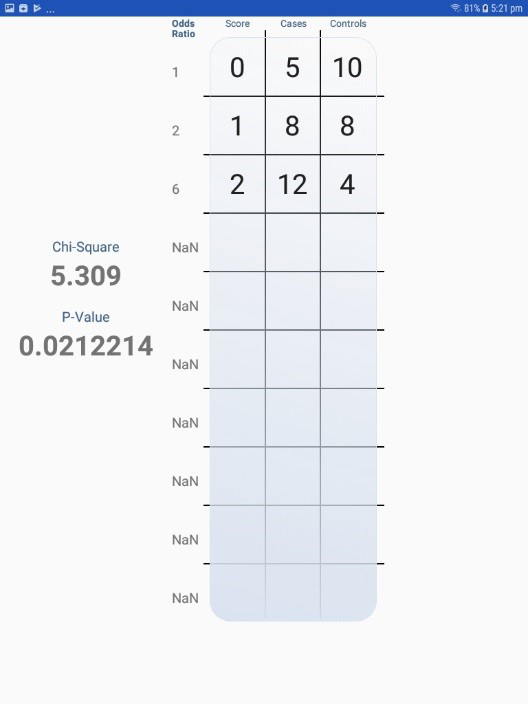Figure 6.76: Analysis of Linear Trend

### Poisson

The Poisson distribution states the probability that a number of positive outcomes occurs based on the expected number of positive outcomes. To analyze the Poisson distribution, enter the expected number of positive outcomes in Expected # of events and the value of positive outcomes you would like to determine the probability of in Observed # of events.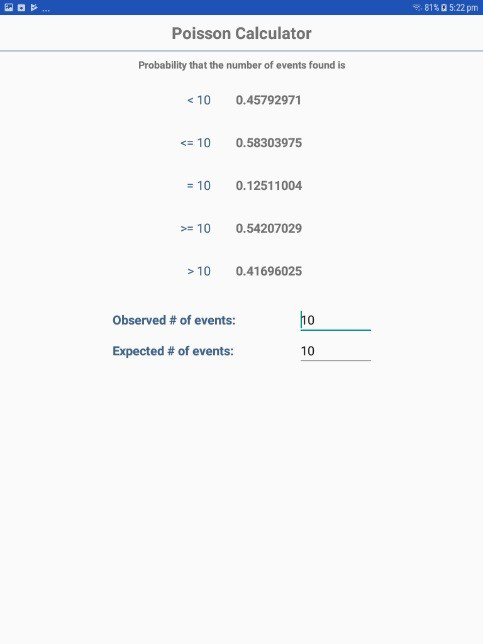Figure 6.77: Poisson Distribution

### Binomial

The binomial distribution states the probability that a number of positive outcomes occurs given the expected percentage of positive outcomes and the total number of observations taken.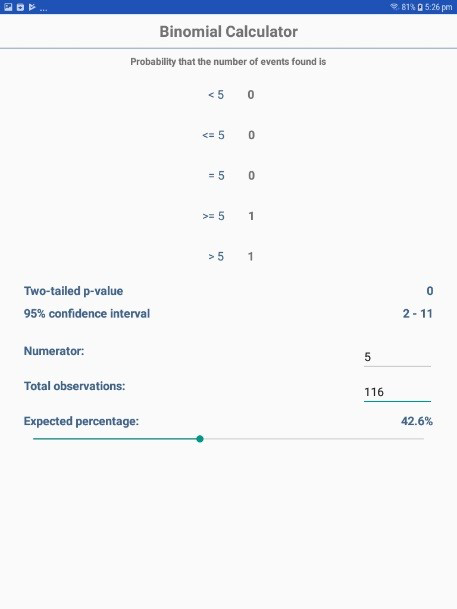Figure 6.78: Binomial Distribution

### OpenEpi.com

The OpenEpi.com website is an open source web tool that provides additional epidemiologic statistics.Figure 6.79: OpenEpi.com

Page last reviewed: September 16, 2022, 12:00 pm